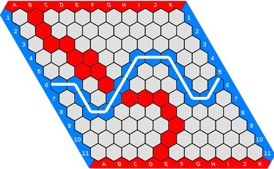# CTYI Game Theory Information

"We often hear that mathematics consists mainly of 'proving theorems'. Is a writer's job mainly that of 'writing sentences'?" - Gian-Carlo Rota.Here is the course outline. Here is our Handbook that we used for the course.
Here is the Grade Game Handout that we spoke about in class (the one from Yale).
Here is the mixed strategies handout that we spoke about in class (the one from Yale).
Here's the Game Theory Handout regarding mixed strategy Nash equilibria & how to calculate them for the weekend. Have a read and ask me questions!
Finally, here's a blank Hex board courtesy of Wikipedia.
Image credit; Wikipedia.

When we spoke about the game Order and Chaos, we used the following material:
Solving Order and Chaos, a paper demonstrating from an empty board, perfect play on both sides leads to an Order win.
The Order and Chaos webapp, giving the strategy for an Order win.

The mathematical theory for the game of Nim.

When we spoke about the game Bagh-Chal, we used the following material: